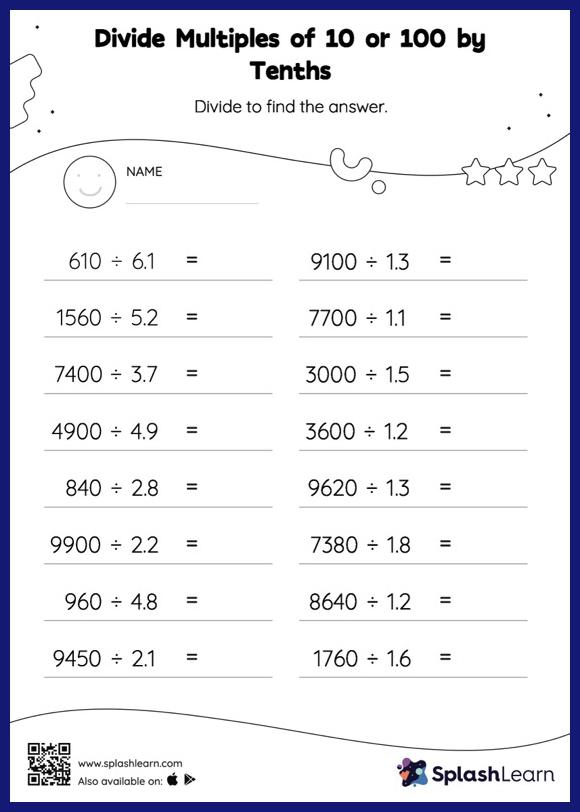# Divide Multiples of 10 or 100 by Tenths without Remainder: Horizontal Division Worksheet

Home > Divide Multiples of 10 or 100 by Tenths without Remainder: Horizontal DivisionGive your little one the opportunity to dive deeper into division with this divide multiples of 10 or 100 by tenths without remainder worksheet. When dividing a number by a decimal, students divide without considering the decimal point and then move each digit of the quotient to the left based on the number of decimal digits in the divisor. Divide multiples of 10 or 100 by tenths without remainder provides plenty of practice with this concept.